## Byte Introduction

Dive into the function call stack and surface with a better understanding of it

Skills:

OS Concepts

#### Objective

Understand how the function call variables are represented and stored on the stack.

#### Prerequisite

Taking up the "Fun with Process Internals" Byte will be useful in understanding this Byte better.

#### Background

Process stack and heap are foundational concepts in computer science. Whenever a process runs, its memory is organized into a bunch of segments including heap and stack.

Recall the below diagram for the layout of process memory.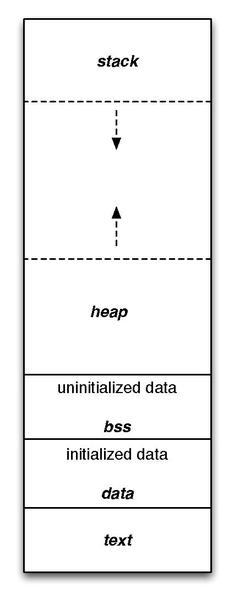Stack

The stack area contains the program (function call) stack, a LIFO structure, typically located in the higher parts of memory. A "stack pointer" register tracks the top of the stack; it is adjusted each time a value is "pushed" onto the stack. The set of values pushed for one function call is termed a "stack frame" or an "activation record". A stack frame consists at minimum of a return address. Automatic/local variables are also allocated on the stack.

Stack Frame / Activation Records

Each stack frame corresponds to a call to a subroutine which has not yet terminated with a return. For example, if a subroutine named DrawLine is currently running, having been called by a subroutine DrawSquare, the top part of the call stack might be laid out like in the below picture.

The stack frame at the top of the stack is for the currently executing routine (the stack pointer would be pointing here). The stack frame usually includes at least the following items (in push order):

• the arguments (parameter values) passed to the routine (if any);

• the return address back to the routine's caller (e.g. in the DrawLine stack frame, an address into DrawSquare's code); and

• space for the local variables of the routine (if any).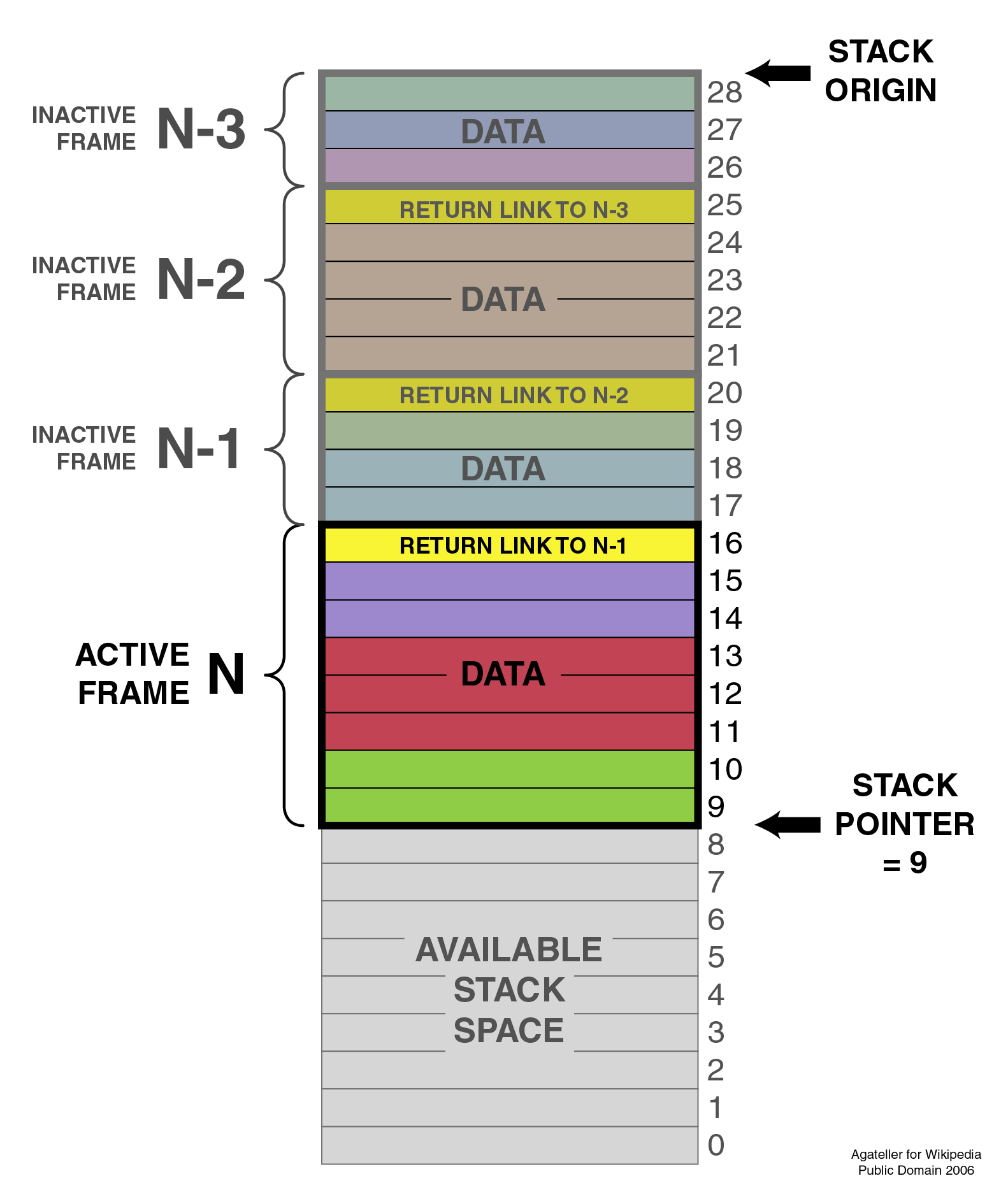##### Layout of an activation record in the stack

The active frame is the function that is currently in execution. You will understand the Data section of an activation record in much higher detail as you go through the tasks.

#### Primary goals

1. Understand the basic structure of a process stack (function call stack) and the way it gets used

2. Understand the layout of an activation record (stack frame)

#### Objective

Understand how the function call variables are represented and stored on the stack.

#### Prerequisite

Taking up the "Fun with Process Internals" Byte will be useful in understanding this Byte better.

#### Background

Process stack and heap are foundational concepts in computer science. Whenever a process runs, its memory is organized into a bunch of segments including heap and stack.

Recall the below diagram for the layout of process memory.Stack

The stack area contains the program (function call) stack, a LIFO structure, typically located in the higher parts of memory. A "stack pointer" register tracks the top of the stack; it is adjusted each time a value is "pushed" onto the stack. The set of values pushed for one function call is termed a "stack frame" or an "activation record". A stack frame consists at minimum of a return address. Automatic/local variables are also allocated on the stack.

Stack Frame / Activation Records

Each stack frame corresponds to a call to a subroutine which has not yet terminated with a return. For example, if a subroutine named DrawLine is currently running, having been called by a subroutine DrawSquare, the top part of the call stack might be laid out like in the below picture.

The stack frame at the top of the stack is for the currently executing routine (the stack pointer would be pointing here). The stack frame usually includes at least the following items (in push order):

• the arguments (parameter values) passed to the routine (if any);

• the return address back to the routine's caller (e.g. in the DrawLine stack frame, an address into DrawSquare's code); and

• space for the local variables of the routine (if any).##### Layout of an activation record in the stack

The active frame is the function that is currently in execution. You will understand the Data section of an activation record in much higher detail as you go through the tasks.

#### Primary goals

1. Understand the basic structure of a process stack (function call stack) and the way it gets used

2. Understand the layout of an activation record (stack frame)

#### Getting Started

• You would need a Linux machine with sudo access.

• Have a g++ compiler to run simple cpp programs.

``````
g++ SampleProgram.cc -o SampleProgram

./SampleProgram

``````
• Understand how to run a process in the background and get its process id.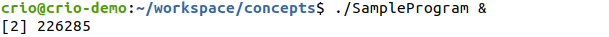When you run a program with ‘&’ in the end, it runs as a background job and prints the process id. In the above case, 226285 is the process id.

• You may have to periodically kill these processes you put in the background. Otherwise your system may become slow. If you run `ps` in the same terminal, you will be able to see the list of all processes. You can then kill the process either using pkill or kill commands.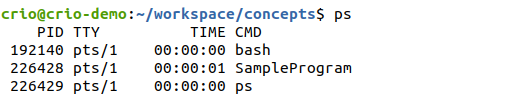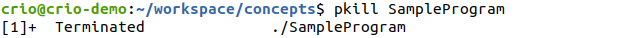#### Where do function variables get stored?

Start with a simple C++ program to understand how a process memory is typically laid out. Run the following program and get it’s process id.

``````
// File: FunctionCallStack.cc

#include <iostream>

using namespace std;

void function1() {

int funtion1_variable;

cout << "Address of function1 variable: " << &funtion1_variable << endl;

}

int main() {

cout << endl << "Let's Learn by Doing!" << endl;

int stack_variable;

cout << "Address of stack variable: " << &stack_variable << endl;

int *ptr_heap = new int;

cout << "Address of heap: " << ptr_heap << endl;

function1();

// Infinite loop to keep the process running for you to examine the procfs.

while (1) {}

}

``````

You would see something like this: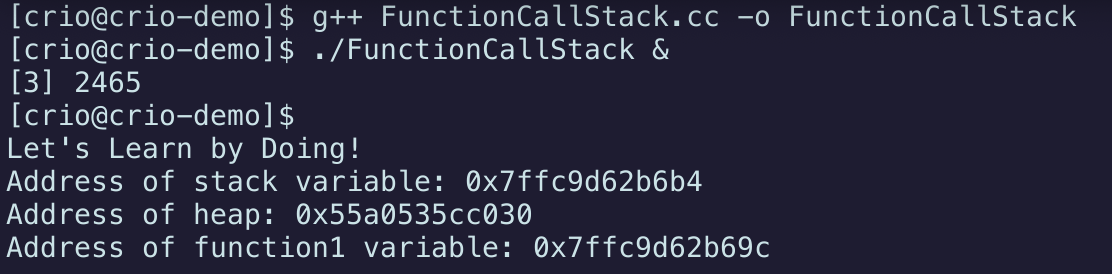Now, check the proc maps output for this process to look at the stack and heap segment ranges.

To do this, you can pick up the process id shown after the function was executed (as seen above) or find the process id of the running FunctionCallStack process using the `ps` command. Then run `cat /proc/[process id]/maps`.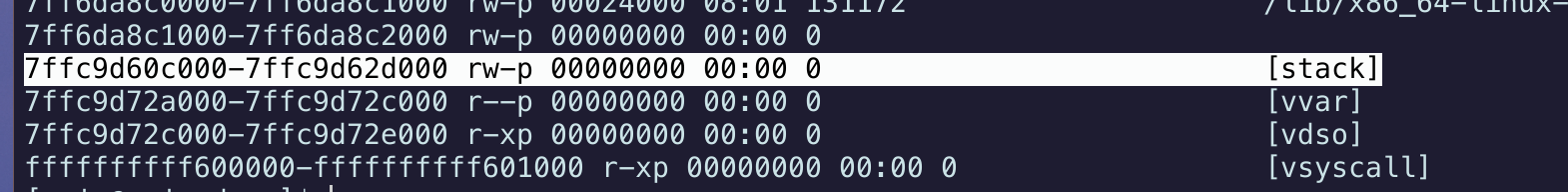Here you can see that Stack variables are between 0x7ffc9d60c000 - 0x7ffc9d60c000 and we can also see that -> 0x7ffc9d60c000 < 0x7ffc9d62b69c (function1_variable) > 0x7ffc9d60c000.

This confirms that function variables are allocated within the stack.

### References

#### Body Double

Now let’s try adding another function call and see what happens.

``````
// File: FunctionCallStackConsecutive.cc

#include <iostream>

using namespace std;

void function1() {

int funtion1_variable;

cout << "Address of function1 variable: " << &funtion1_variable << endl;

}

void function2() {

int function2_variable;

cout << "Address of function2 variable: " << &function2_variable << endl;

}

int main() {

cout << endl << "Let's Learn by Doing!" << endl;

int stack_variable;

cout << "Address of stack variable: " << &stack_variable << endl;

int *ptr_heap = new int;

cout << "Address of heap: " << ptr_heap << endl;

function1();

function2();

// Infinite loop to keep the process running for you to examine the procfs.

while (1) {}

}

``````

You may see a similar output when you run the program: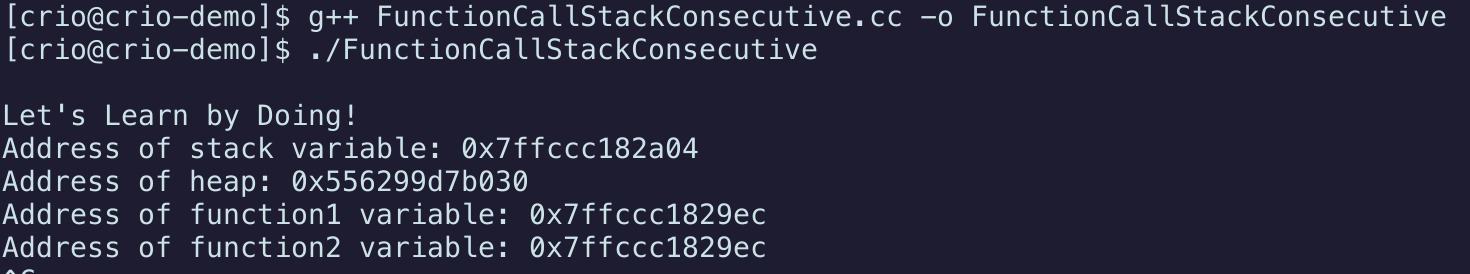Wait! Did you see that? - function1 and function2 variables are pointing to the same memory location.

#### Curious Cats

What just happened here? Can you explain this? Hint - function1() had already returned by the time function2() was invoked. Picture the stack.

#### Local Static Variables

Modify the program from the previous milestone to allocate a static variable within function1. Syntax as below

``````
static data_type var_name = var_value;

``````

Print the address of var_name and find out the range that it is allocated to.

### References

#### Curious Cats

• Is there a difference between where an initialized static variable is stored vs an uninitialized static variable in the process memory map?

• How do different languages handle static variables?

#### Accessing variables from other functions. Can you do it?

Using the following program, can you try to access the value of function1_data from function2 (without passing it as a parameter to function2 of course :p)?

The `hexdhump.hpp` file can be downloaded from here. The Hexdump function can be used to print the values of addresses starting from a pointer till a specified range.

Try printing the values of pointers near funtion2_data and see if you are able to access variables from other functions.

``````
// File: CrossFunctionAccess.cc

#include <iostream>

#include <cstring>

#include "hexdump.hpp"

using namespace std;

void function2() {

unsigned char function2_data[] = "!Doing!";

int size = sizeof(function2_data);

cout << "Address of function2_data: " << &function2_data << endl;

cout << Hexdump(function2_data, size) << endl;

//cout << /* try to print value of function1_data here */

}

void function1() {

char function1_data[] = "Learn by";

cout << "Address of function1_data: " << &function1_data << endl;

function2();

}

int main() {

cout << endl << "Let's Learn by Doing!" << endl;

int stack_variable;

cout << "Address of stack variable: " << &stack_variable << endl;

function1();

}

``````

Output should look like this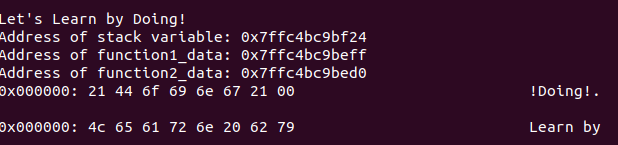#### Can you access the function parameter value without using the argument variable?

Try to figure out how to print the function parameter value without using the variable that was passed directly.

If you try to print the hexdump similar to the previous task, you can see that the function parameter value is not visible.

Do you need to print the bytes from the previous addresses? Visualize the stack.

hexdhump.hexdhump.hexdhump.

We promise a hint if you are stuck :)

``````
// File: MessWithFunctions.cc

#include <iostream>

using namespace std;

void function1(int function1_argument) {

int function1_variable = 5;

cout << "Address of function1_variable: " << &function1_variable << endl;

cout << "Address of function1_argument: " << &function1_argument << endl;

//cout << "Value of function1_argument: " << /* TODO: fill in here  */ << endl;

}

int main() {

cout << endl << "Let's Learn by Doing!" << endl;

int input = 2;

function1(input);

}

``````

#### Newfound Superpowers

• You are now confident (probably more than the people who designed it ;)) that local variables and function arguments are stored in the stack within each function’s stack frame/activation record.

But is that all?

There’s a little more to it as you can see from the diagram below but this is a good start for us to get a general idea of how things work in the call stack.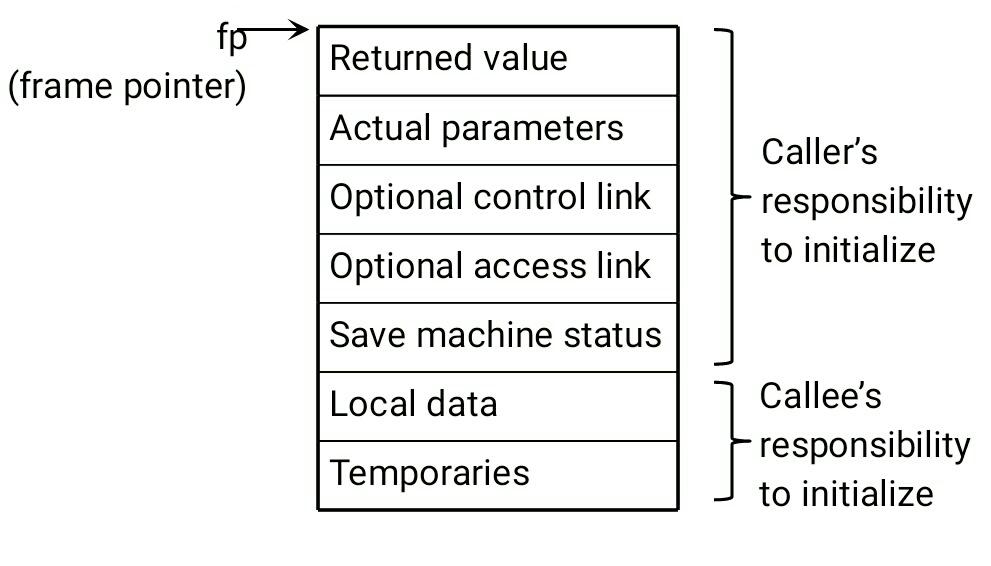#### Now you can

1. Correlate and visualize what people mean by a stack trace or call stack when they talk about debugging.

2. Understand how the program is able to print the stack trace at run-time, when there’s an issue.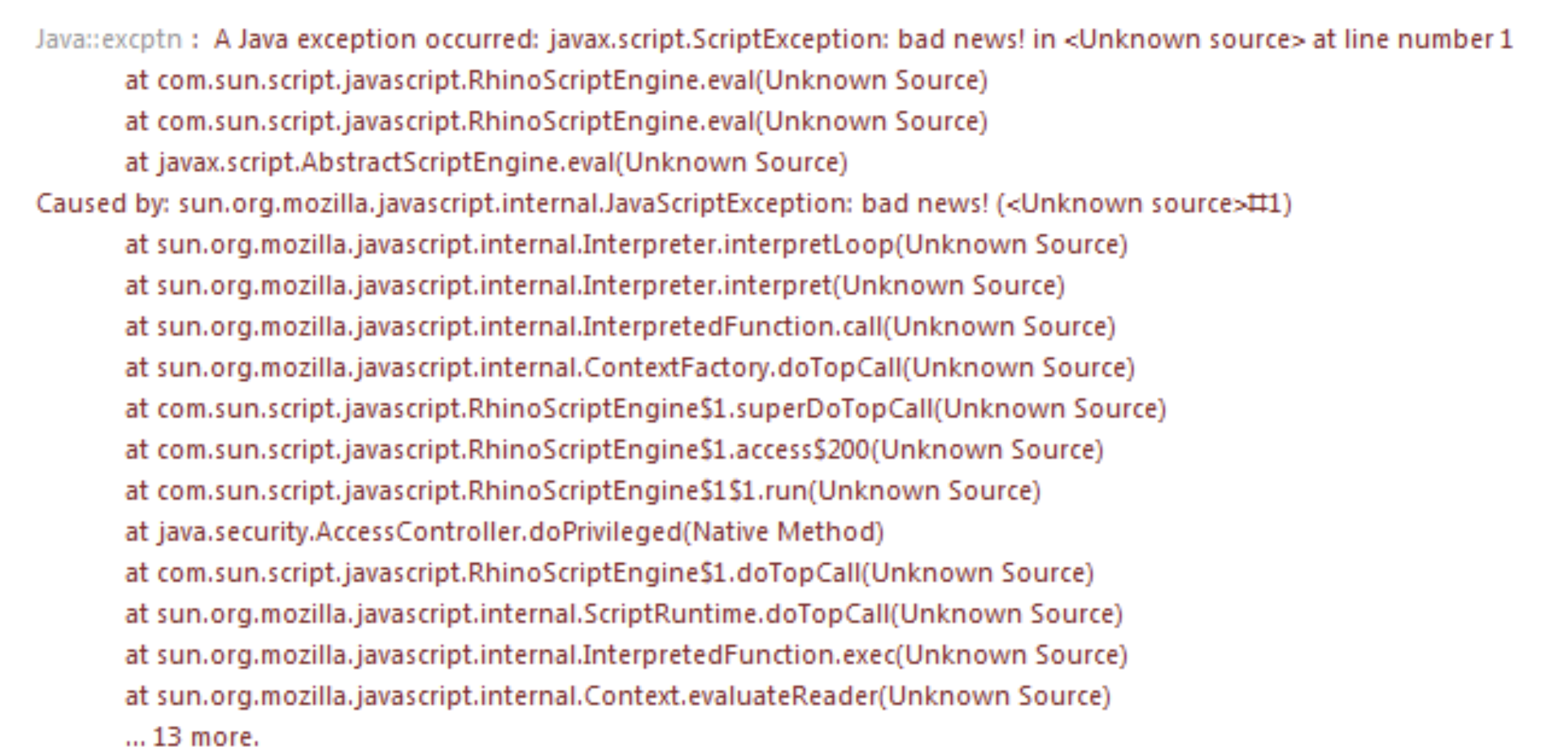The system is able to step through the activation records/stack frames and collect the necessary information and dump the data as shown above. This gives us the function call hierarchy that led to the failure.

Crazy, right? Who knew this much effort was needed for the program to just keep track of what function is currently executing and making sure the control gets returned to the right function!

#### Curious Cats

• Do you know what Control Link and Access Link in an Activation Record mean?

• When you attach a debugger to a program, will you be able to look at any function on the function call stack and its variables? Give that a shot. This skill is very much a part of the software engineer’s arsenal.